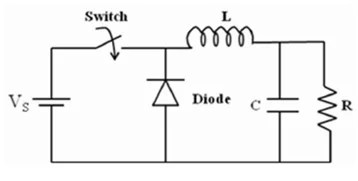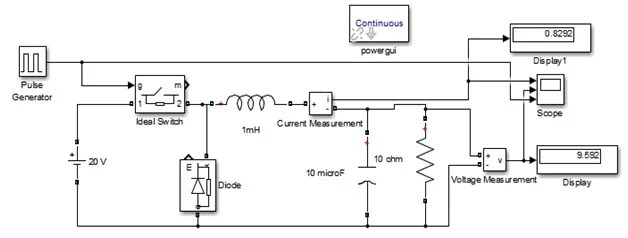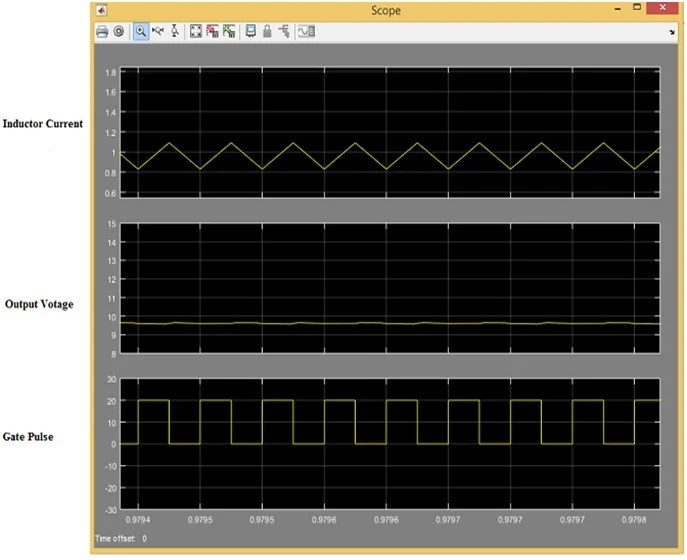# Dc to dc buck converter simulation with Simulink

DC to DC Buck Converter simulation with MATLAB Simulink Model: Dc to dc buck converter is a converter in which dc voltages are step down to desired level by high frequency switching of semiconductor switches such as MOSFET or IGBTs. This type of converter is also called step down converter. If we talk about regulated supply then it is not so much difficult in ac side but in dc side it is so much difficult and this is only possible with high frequency switching of semiconductor switches. This type of converter is mainly used in switch mode power supplies and dc motor control system. The schematic diagram of buck converter is shown in figure 1Figure 1 The Schematic Diagram of Buck Converter

### Working Principle of DC to DC Buck Converter simulation

This dc to dc buck converter works in two states, one when switch is closed and second one when switch is opened. When switch is in close state then diode becomes reverse biased then input source provides the energy to inductor as well as output load. Similarly, when switch is in open state then inductor becomes discharged through diode and transfers some of its stored energy to output load. Because the switch is open and close at high frequency therefore higher order of harmonic are generate which are filtered through low pass filter.

For on or off the switch at high frequency PWM (pulse width modulation) technique is used. The duty cycle of this PWM is calculated by the formula,Where D is duty cycle, Vo is output voltage and Vin is input voltage.We can see that the output voltage is directly proportional to duty cycle D means which duty ratio is increased then output voltage is also increased as well as when duty cycle is decreased then output voltage is also decreased. We will prove this by using MATLAB Simulink model. The Simulink model has been defined below.

### MATLAB Simulink Model of Dc to Dc Buck Converter simulationFigure 2 MATLAB Simulink Model of Dc to Dc Buck Converter simulation

In this MATLAB Simulink model, an ideal switch is used for regulating the 20V dc voltages in to 10V dc. The ideal switch is on or off through pulse generator which gives the 20V amplitude switching pulse of 20KHz frequency at 50% duty ratio which is gained by the duty ratio formula. The output of this converter is not pure dc it contains the high frequency harmonic content which are removed through low pass filter. In this model 1mH inductor and 10μF capacitor has been used such as low pass filter.10-ohm resistor has been used such as an output load. When this model is simulated in MATLAB then we can see that it shows 9.562 output voltage and .8292 inductor current. Which are showed in below simulation results.

### Video of simulation

Dc to Dc Buck Converter Simulation Results:

Inductor current, output voltage and switching pulse of dc to dc buck converter is shown below in figure 3,Figure 3 Inductor Current, Output Voltage and Switching Pulse of DC to DC Buck Converter

Every dc to dc converter works in two modes of operation, one is CCM (continuous conduction mode), in this mode inductor current never goes to zero and second one is DCM (discontinuous conduction mode), in this mode inductor current goes to zero at every switching pulse. Here we can see that in figure 3 this dc to dc buck converter works in CCM mode because inductor current never goes to zero. When switching pulse is positive then inductor is charged at its peak value during this, the output capacitor continuously provides the voltage to output load. Similarly, when switch pulse is negative then this inductor is discharged through diode and charge the capacitor. This process is continued at every switch pulse of dc to dc buck converter.